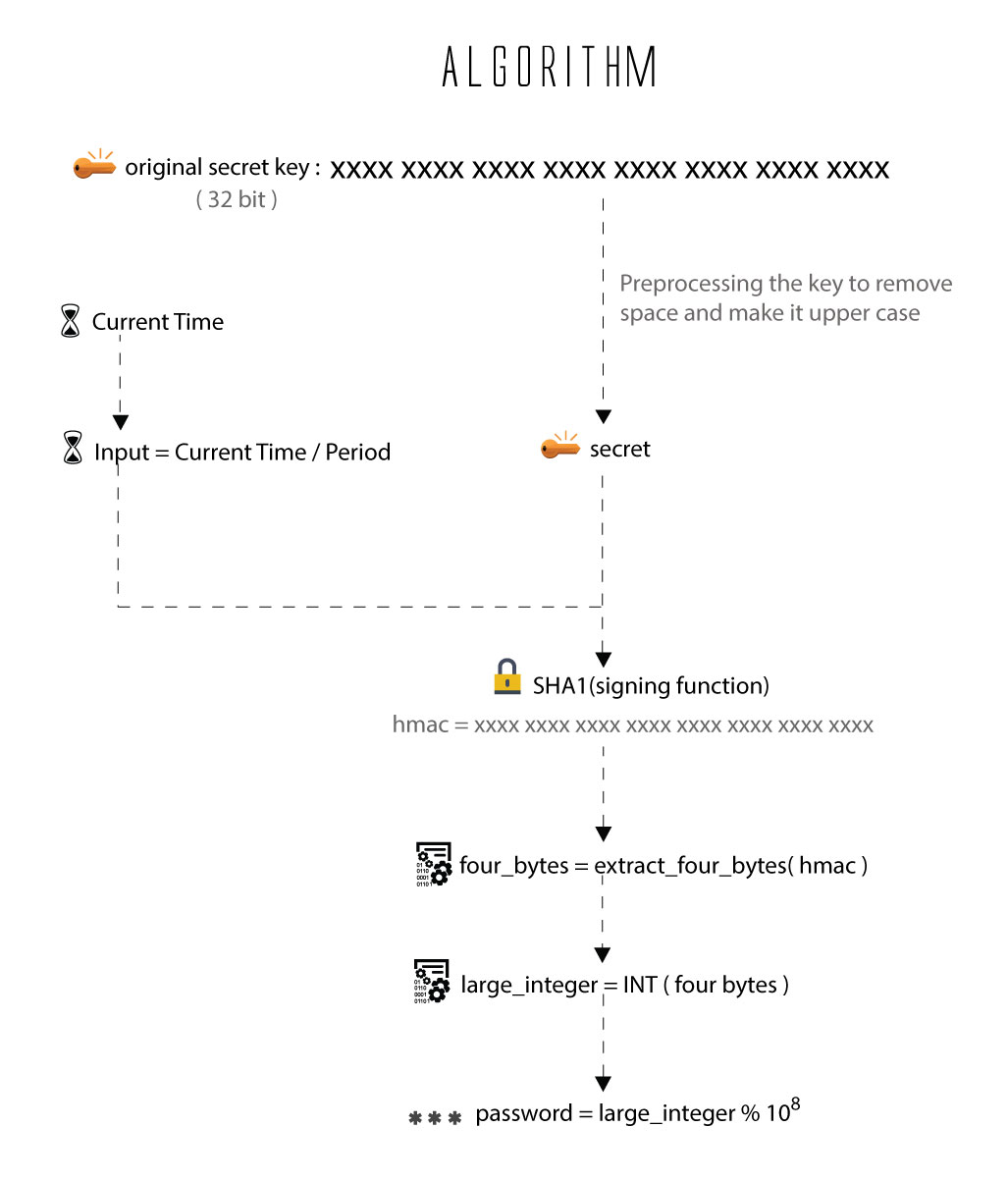• 由服务器和工具生成密码，无须记录。
• 随机生产，一次有效性。
• 根据时间计算，有时效性。

## 动态密码的分类

• 计次使用：计次使用的OTP产出后，可在不限时间内使用，知道下次成功使用后，计数器加 1，生成新的密码。用于实现计次使用动态密码的算法叫 HOTP，接下来会对这个算法展开介绍；
• 计时使用：计时使用的OTP则可设定密码有效时间，从30秒到两分钟不等，而OTP在进行认证之后即废弃不用，下次认证必须使用新的密码。用于实现计时使用动态密码的算法叫 TOTP，接下来会对这个算法展开介绍。

## HOTP

HOTP 算法，全称是“An HMAC-Based One-Time Password Algorithm”，是一种基于事件计数的一次性密码生成算法，详细的算法介绍可以查看 RFC 4226

HOTP(K,C) = Truncate(HMAC-SHA-1(K,C))

PWD(K,C,digit) = HOTP(K,C) mod 10^Digit


• K 代表我们在认证服务器端以及密码生成端（客户设备）之间共享的密钥，在 RFC 4226 中，作者要求共享密钥最小长度是 128 位，而作者本身推荐使用 160 位长度的密钥
• C 表示事件计数的值，8 字节的整数，称为移动因子（moving factor），需要注意的是，这里的 C 的整数值需要用二进制的字符串表达，比如某个事件计数为 3，则C是 "11"（此处省略了前面的二进制的数字0）
• HMAC-SHA-1 表示对共享密钥以及移动因子进行 HMAC 的 SHA1 算法加密，得到 160 位长度（20字节）的加密结果,当然也可以使用HMAC-MD5等算法。
• Truncate 即截断函数，后面会详述
• digit 指定动态密码长度，比如我们常见的都是 6 位长度的动态密码

### Truncate 截断函数

DT(String) // String = String…String

Let OffsetBits be the low-order 4 bits of String
Offset = StToNum(OffsetBits) // 0 <= OffSet <= 15
Let P = String[OffSet]...String[OffSet+3]
Return the Last 31 bits of P


先从第一步通过 SHA-1 算法加密得到的 20 字节长度的结果中选取最后一个字节的低字节位的 4 位（注意：动态密码算法中采用的大端(big-endian)存储）；



## TOTP

TOTP 算法，全称是 TOTP: Time-Based One-Time Password Algorithm，其基于 HOTP 算法实现，核心是将移动因子从 HOTP 中的事件计数改为时间差。完整的 TOTP 算法的说明可以查看 RFC 6238，其公式描述也非常简单：

TOTP = HOTP(K, T)

// T is an integer and represents the number of time steps between the initial counter time T0 and the current Unix time More specifically,
T = (Current Unix time – T0) / X, where the default floor function is used in the computation.


{# /images/otp.jpg #}## code by golang

package otp

import (
"crypto/hmac"
"crypto/sha1"
"encoding/base32"
"encoding/binary"
"fmt"
"net/url"
"time"
)

//
// OTP(K,C) = Truncate(HMAC-SHA-1(K,C))
//
func OTP(secret string, counter int64) int {
key, err := base32.StdEncoding.DecodeString(secret)
if err != nil {
return -1
}

hash := hmac.New(sha1.New, key)
err = binary.Write(hash, binary.BigEndian, counter)
if err != nil {
return -1
}
hs := hash.Sum(nil)

offset := hs & 0x0f

truncated := binary.BigEndian.Uint32(hs[offset : offset+4])

truncated &= 0x7fffffff
code := truncated % 1000000

return int(code)

}

// HOTP ...
type HOTP struct {
secret string
digits int
size   int // 3-5 验证范围 totp 时候追加验证的范围
}

// At 生成
func (h HOTP) At(counter int64) int {
return OTP(h.secret, counter)
}

// Verify 验证 OTP code
func (h HOTP) Verify(code int, counter int64) bool {
return h.At(counter) == code
}

// NewHOTP 生成 HOTP instance
func NewHOTP(secret string, digits int) (h *HOTP) {
h = new(HOTP)
h.secret = secret
h.digits = digits
return
}

//-------------------

// TOTP 基于时间戳的双因子验证
type TOTP struct {
secret string
digits int
x      int64 // 设定有效期，建议 30 ，30秒
UTC    bool
}

// NewTOTP generate new TOTP instance
// digits 位数
// x 时间有效期 秒
func NewTOTP(secret string, digits, x int) (t *TOTP) {
t = new(TOTP)
t.secret = secret
t.digits = digits
t.x = int64(x)
return t
}

// At 获取时间因子 OTP code
func (t *TOTP) At(timestamp int64) int {
// 用时间戳当做算法的移动因子, 除去有效实际
counter := int64(timestamp / t.x)
return OTP(t.secret, counter)
}

// Now 获取当前时间 TOTP
func (t *TOTP) Now() int {
now := time.Now()
timestamp := now.Unix()
return t.At(timestamp)
}

// VerifyNow 验证当前时间 OTP code
func (t *TOTP) VerifyNow(code int) bool {
return t.Now() == code
}

// Verify 验证 OTP code
func (t *TOTP) Verify(code int, timestamp int64) bool {
return t.At(timestamp) == code
}

// URI 生成 Google Authenticator 字符串
// 用于二维码生成
func (t *TOTP) URI(user string, issuer string) string {
auth := "totp/"
q := make(url.Values)

// if HotpCounter > 0 {
// 	auth = "hotp/"
// }

if issuer != "" {
auth += issuer + ":"
}

return "otpauth://" + auth + user + "?" + q.Encode()

}

// ScratchCode 救援码验证
func (t *TOTP) ScratchCode(Scratch ScratchCode, code int) bool {
return Scratch.Verify(code)
}

// ScratchCode 救援码
type ScratchCode interface {
Verify(code int) bool // 救援码验证
Generate() []string   // 救援吗生成
}

// Scratch 救援码
type Scratch struct {
ScratchCodes []int
}

// Generate 救援吗生成
func (s *Scratch) Generate(code ...int) {
s.ScratchCodes = append(s.ScratchCodes, code...)
}

// Verify 救援码 ？？ 修改为数据。。。
func (s *Scratch) Verify(code int) bool {
for i, v := range s.ScratchCodes {
if code == v {
// 存在则删除
l := len(s.ScratchCodes) - 1
s.ScratchCodes[i] = s.ScratchCodes[l]
s.ScratchCodes = s.ScratchCodes[0:l] // 复制(重置长度)
return true
}
}
return false
}